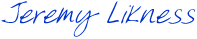I noticed a project on CodePlex that provides a “JQuery-like syntax” for parsing XAML. It looked interesting, but then I wondered why it would be needed when we have such powerful features available to us in the existing runtime. Silverlight provides a powerful `VisualTreeHelper` for iterating elements in the visual tree. It also provides a `TransformToVisual` function to find the offset of elements relative to other elements.

So first things first: create a function that parses all children in the visual tree. You can either parse specific types of children, or all children that derive from a type and then filter or group on those. The following snippet will handle this:

```public static IEnumerable<T> RecurseChildren<T>(DependencyObject root) where T: UIElement
{
if (root is T)
{
yield return root as T;
}

if (root != null)
{
var count = VisualTreeHelper.GetChildrenCount(root);

for (var idx = 0; idx < count; idx++)
{
foreach (var child in RecurseChildren<T>(VisualTreeHelper.GetChild(root, idx)))
{
yield return child;
}
}
}
}
```

So why enumerable? Because sometimes we may want to only get the first item in the list. By making this enumerable, the iterator will stop once the query is done, avoiding the work of recursing n-levels deep. Using some simple LINQ functions, we can now get the first text box in the visual tree:

```var firstTextBox = RecurseChildren<TextBox>(Application.Current.RootVisual).FirstOrDefault();
```

Of course, we might want to parse all of the text boxes:

```var textboxes = RecurseChildren<TextBox>(Application.Current.RootVisual).ToList();
```

Let’s try something more interesting. Let’s say you have two list boxes and want to move text from one to the other. In order to animate the text blocks, you’ll need to put a text block in the main visual tree (outside of the list box) but overlay the list box and then animate to the other list box. How do you get the offset of the text block relative to the list box? Use the visual transform helper. If your list box is referenced as “listBox” then the following will generate a list of tuples that contain TextBlocks and the offset of the upper left corner of the TextBlock from the upper left corner of the ListBox:

```var textBlockOverlays = from tb in RecurseChildren<TextBlock>(Application.Current.RootVisual)
let offsetFromListBox = tb.TransformToVisual(listBox).Transform(new Point(0, 0))
select Tuple.Create(tb, offsetFromListBox);
```

Now let’s have a lot of fun. What about creating a rectangle that overlayss all text retuned by your query? What we need to do is get the upper left and lower right bounds for each text block using the offset from the origin and the width and height, then use a minimum and maximum function to create the rectangle, for example:

```var bounds = (from o in
(from tb in RecurseChildren<TextBlock>(Application.Current.RootVisual)
let upperLeft =
tb.TransformToVisual(Application.Current.RootVisual).Transform(new Point(0, 0))
select Tuple.Create(upperLeft, new Point(upperLeft.X + tb.ActualWidth,
upperLeft.Y + tb.ActualHeight)))
group o by 1
into g
let minX = g.Min(o => o.Item1.X)
let maxX = g.Max(o => o.Item2.X)
let minY = g.Min(o => o.Item1.Y)
let maxY = g.Max(o => o.Item2.Y)
select new Rect(new Point(minX, minY), new Point(maxX, maxY))).FirstOrDefault();
```

As you can see, the possibilities are endless … you can easily check for intersections between elements and find any type of element no matter how deep within the tree it is. This will work whether you have nested user controls, content controls, panel items or anything else that can render in the visual tree.